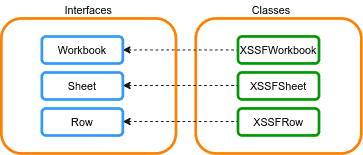### Get started with Spring 5 and Spring Boot 2, through the Learn Spring course:

>> CHECK OUT THE COURSE

## 1. Overview

Sometimes, we might need to manipulate Excel files in a Java application.

In this tutorial, we’ll look specifically at inserting a new row between two rows in an Excel file using the Apache POI library.

## 2. Maven Dependency

First, we have to add the poi-ooxml Maven dependency to our pom.xml file:

``````<dependency>
<groupId>org.apache.poi</groupId>
<artifactId>poi-ooxml</artifactId>
<version>5.2.0</version>
</dependency>``````

## 3. Inserting Rows Between Two Rows

Apache POI is a collection of libraries — each one dedicated to manipulating a particular type of file. The XSSF library contains the classes for handling the xlsx Excel format. The figure below shows the Apache POI related interfaces and classes for manipulating xlsx Excel files:### 3.2. Implementing the Row Insert

For inserting m rows in the middle of an existing Excel sheet, all the rows from the insertion point to the last row should be moved down by m rows.

First of all, we need to read the Excel file. For this step, we use the XSSFWorkbook class:

``Workbook workbook = new XSSFWorkbook(fileLocation);``

The second step is accessing the sheet in the workbook by using the getSheet() method:

``Sheet sheet = workbook.getSheetAt(0);``

The third step is shifting the rows, from the row currently positioned where we want to begin the insertion of new rows, through the last row of the sheet:

``````int lastRow = sheet.getLastRowNum();
sheet.shiftRows(startRow, lastRow, rowNumber, true, true);
``````

In this step, we get the last row number by using the getLastRowNum() method and shift the rows using the shiftRows() method. This method shifts rows between startRow and lastRow by the size of rowNumber.

Finally, we insert the new rows by using the createRow() method:

``sheet.createRow(startRow);``

It’s worth noting that the above implementation will keep the formatting of the rows being moved. Also, if there are hidden rows in the range we’re moving, they move during the insertion of new rows.

### 3.3. Unit Test

Let’s write a test case that reads a workbook in the resource directory, then inserts a row at position 2 and writes the content to a new Excel file. Finally, we assert the row number of the result file with the main file.

Let’s define a test case:

``````public void givenWorkbook_whenInsertRowBetween_thenRowCreated() {
int startRow = 2;
int rowNumber = 1;
Workbook workbook = new XSSFWorkbook(fileLocation);
Sheet sheet = workbook.getSheetAt(0);

int lastRow = sheet.getLastRowNum();
if (lastRow < startRow) {
sheet.createRow(startRow);
}

sheet.shiftRows(startRow, lastRow, rowNumber, true, true);
sheet.createRow(startRow);

FileOutputStream outputStream = new FileOutputStream(NEW_FILE_NAME);
workbook.write(outputStream);

File file = new File(NEW_FILE_NAME);

final int expectedRowResult = 5;
Assertions.assertEquals(expectedRowResult, workbook.getSheetAt(0).getLastRowNum());

outputStream.close();
file.delete();
workbook.close();
}``````

## 4. Conclusion

In summary, we’ve learned how to insert a row between two rows in an Excel file using the Apache POI library. As always, the full source code of the article is available over on GitHub.

### Get started with Spring 5 and Spring Boot 2, through the Learn Spring course:

>> CHECK OUT THE COURSE